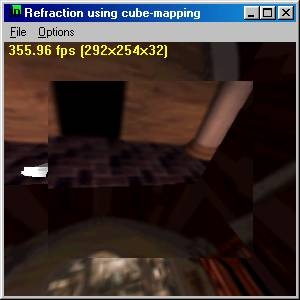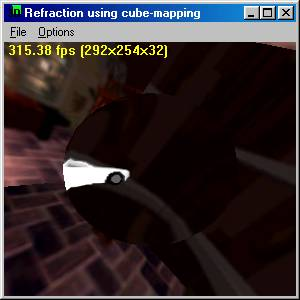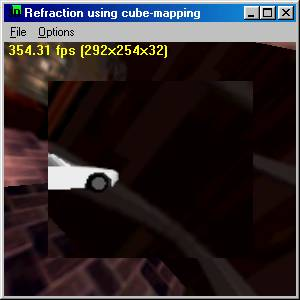# Fake Refraction in DX7

Back when the Geforce 1 first came out (around May, 2000) I wrote a demo on how to use cube-maps to fake refractions.

There is a paper is available in word document or PDF file format.

## Approximating Refraction

Problem Statement

Refraction is a phenomenon that simulates the bending of light rays through semi-transparent objects.  Refraction can make a 3d graphics scene look much more realistic.  Real world objects can both reflect and refract light waves to distort images seen through them.  There are several properties defined with refraction, Snell's law and the critical angle.  Snell's law states that for a light ray going from a less dense medium to a higher dense medium, the light ray will bend in one direction and going from a higher density medium to a lower density medium, it will bend in the other direction.  These properties are known as the refraction index and it is a ratio of the speed of light through one medium divided by the speed of light through the other medium.

## Snell's Law

Snell's law states that a light is traveling low index to a high index (e.g. air is a low index and glass is a high index), the light ray will be bent toward the normal.  On the other hand if light is traveling from a high index to a low index (e.g. glass to air), the light will bend away from the normal.  Here is a diagram of Snell's law.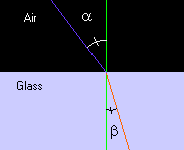The purple line represents a light ray coming hitting a glass object and you can see that according to Snell's law that the light would bend towards the normal in this case (the red line).

Mathematically speaking Snell's law is stated as:

n1 * sin(a) = n2 * sin(b)  where n1 and n2 are the index of refraction for each medium.

If you reverse the sense of the light direction, you can see that if the light were the red line and the light were traveling from the glass to air, it would bend away from the normal of the surface.

Snell also stated that the angles between the incident ray and the normal of the surface and the outgoing ray's angle in regards to that normal could be expressed in terms of a ratio.  This ratio is specified as the speed of light through the medium as compared to the speed of light through a vacuum.  If the speed of light through a vacuum is expressed as 1.0, all other mediums are expressed as a ratio of the speed of light compared to a vacuum.  Here is a short list of refraction index of materials

# Refraction Index

Vacuum

1.00000

Air

1.00029

Alcohols

1.32900

Crown Glass

1.53000

Crystal

2.00000

Diamond

2.41700

Emerald

1.57000

Fused Quartz

1.46000

Heavy Flint Glass

1.65000

Glass

1.50000

Ice

1.30900

Quartz

1.64400

Ruby/Sapphire

1.77000

Salt

1.54400

Sugar Solution (80%)

1.49000

Sugar Solution (30%)

1.38000

Topaz

1.61000

Water

1.33333

Snell's law simply states that given an incident ray at an angle relative to the normal of a surface, that ray will change angle based on the refraction index, which is simply a ratio to reduce/increase the angle by after the ray passes from one medium to another.

So if it is assumed that we are going from a vacuum (which is close to the refraction index of air) to water and we know the incident angle and refraction index, we can figure out what the outgoing angle should be. We can rewrite the equation as:

sin(a)  / n2 = sin(b)

One other point is the fact that most rays enter a medium and leave a medium.  For example a ray enters a sheet of glass and then exits the other side of that glass.  In this diagram, the top purple line is the incident ray entering the glass, the red line is the ray refracted in the glass and the bottom purple line is the ray coming out of the glass.As can be seen from the above diagram the light ray actually is parallel to the incoming light ray, but is just moved slightly towards the normal.  On the other hand if the light ray strikes an object that is in water, then transmits to glass and out to air, then that object contributes to the bending of the light rays.

The reason our technique works in the above example is that you can approximate the ray going into the glass and coming out of it by just using on ray as shown in the following diagram: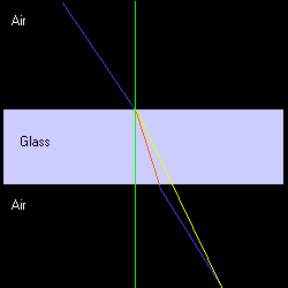The lines above are all the same as in the previous diagram, except for the yellow line. This line represents the refraction index that is actually used since we are just interested in the end point of the ray.

## How DirectX Calculates Reflection Vectors

R = 2(N · E) N - E

Since reflection is stated in terms of the refraction formula:

R = -(a - 1)( (N · E) N - E, where a = index of refraction and a = -1 for reflection, we can substitute the refraction equation back into each vertex and give that value to the hardware for indexing into the cube maps

How to Use Cube-Maps for the Effect

You setup cube maps exactly the same as if you where going to reflect an object.  Now instead of using the flags, D3DTSS_TCI_REFLECTIONVECTOR, you set the D3DTSS_TCI_PASSTHRU flag and calculate the equations for each vertex.  The hardware will interpolate the texture coordinates across the cube map.  The code sample actually doesn't do this, because this is the default state of Direct X.  But if you wanted to set this up you would specify the following:

SetTextureStageState( 0, D3DTSS_TEXCOORDINDEX, D3DTSS_TCI_PASSTHRU | 0);

## Calculating refraction

Below is the code for calculating the refraction:

// eye vector (doesn't need to be normalized)
FLOAT fENX = m_vEyePt.x - pVIn->v.x;
FLOAT fENY = m_vEyePt.y - pVIn->v.y;
FLOAT fENZ = m_vEyePt.z - pVIn->v.z;

FLOAT fNDotE = pVIn->v.nx*fENX + pVIn->v.ny*fENY + pVIn->v.nz*fENZ;
FLOAT fNDotN = pVIn->v.nx*pVIn->v.nx + pVIn->v.ny*pVIn->v.ny + pVIn->v.nz*pVIn->v.nz;
fNDotE *= 1.0f/gRefractIndex;

// refracted vector
pVIn->v.tu = pVIn->v.nx*fNDotE - fENX*fNDotN;
pVIn->v.tv = pVIn->v.ny*fNDotE - fENY*fNDotN;
pVIn->nz = pVIn->v.nz*fNDotE - fENZ*fNDotN;

Notice that above the calculation of 1.0f/gRefractIndex should be adjusted for minification/magnification that happens when a texture is being used that does not map to a 1-to-1 correspondence with the object being texture mapped.

## Suggested Methods

Several papers including Paul Diefenbach's Thesis and early work by Paul Heckbert and Pat Hanahran, suggest using the planes that are refracting the image by use of a projection matrix that changes the angle of view.  One problem that Paul Diefenbach notes is that using these techniques may not be possible on some hardware because moving the projection matrix, precludes the use of the Z-Buffer.  This technique we have described does not suffer from this limitation as it uses cube-maps to render the scene first.  The technique does not modify the projection matrix, so the Z-Buffer can be used with this technique.

It also might be possible to use the projection matrix along with cube maps to automatically calculate out the values to be used in the cube map.  I've looked into this a little, but I didn't see an obvious way to accomplish this.

Enhancements

Real world objects also reflect the environment.  There is also a critical angle refraction that says when the angles falls between a certain point, the refraction turns into reflection.  Another enhancement would be Fresnel effects.  All of these effects can be achieved.  For the reflection portion, you can alpha blend between the refraction effect and the reflection effect.

Limitations

With the current scheme there isn't an easy way to simulate placing a pencil in a glass of water.  This technique also does not allow internal reflections.

## Conclusion

This effect is using an approximation to refraction.  You can achieve real time frame rates using this technique and the end result is visually very good.  Throughout this document you will noticed that I did this from the perspective of ray tracing, but you can also view this as ray casting from the eye point.

Sample - Refraction Index 1.33 (Water)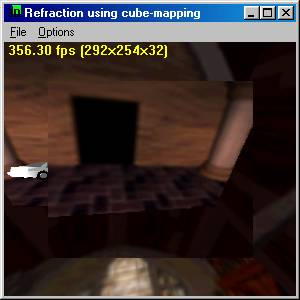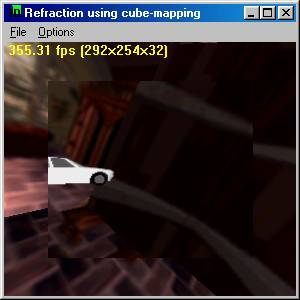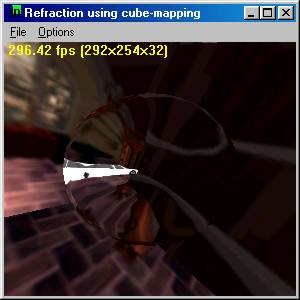Sample - Refraction Index ~= 1.5 (glass)